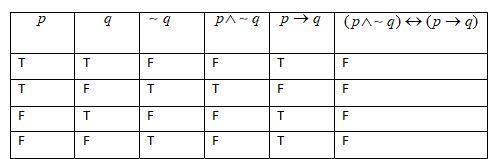Courses
Courses for Kids
Free study material
Free LIVE classes
MoreLIVE
Join Vedantu’s FREE Mastercalss

# Using the truth table, examine whether the following statement pattern is tautology, contradiction and contingency.$(p\wedge \sim q)\leftrightarrow (p\to q)$Verified
363k+ views
Hint: Construct the truth table for $(p\wedge \sim q)\leftrightarrow (p\to q)$ and if all the propositions are true in every row then it is tautology, if all the propositions are false in every row then contradiction and if there is at least one row with true condition and one row with false condition, then it is contingency.Note: $p\wedge q$ is true when both $p$ and $q$ are true. $p\to q$ is true when $q$ is true or both are false. $p\leftrightarrow q$ is true when both $p$ and $q$ are true or both are false. This is the rule used to draw the above truth table.